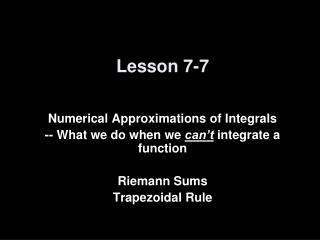DownloadDownload PresentationLesson 7-7

# Lesson 7-7

Télécharger la présentation## Lesson 7-7

- - - - - - - - - - - - - - - - - - - - - - - - - - - E N D - - - - - - - - - - - - - - - - - - - - - - - - - - -
##### Presentation Transcript

1. Lesson 7-7 Numerical Approximations of Integrals -- What we do when we can’t integrate a function Riemann Sums Trapezoidal Rule

2. Strategies for Integrals that we can’t do • Use Riemann Sums – left-hand or right-hand, to approximate the area under the curve (i.e., the value of the definite integral) • Use the Trapezoidal Rule to approximate the area under the curve • The trapezoidal rule gives us an average of the right and left hand Riemann Sum approximations • The graph of fThe trapezoidal rule will: concave down underestimate the area concave up overestimate the area.

3. Trapezoidal Rule • From Geometry: Area of a Trapezoid = ½h•(b1 + b2) • Bases will the “height” or the functional value and h is the width, (b-a)/n, of each trapezoid • Since only the first base and the last base will be used only once  Area = ½h•(b1 + 2b2 + … + 2bn-1 + bn)

4. x=π x=π ∫ sin x dx ∫ sin x dx = 2 x=0 x=0 Example 1 Use the trapezoidal rule with n = 4 to approximate h = (b-a)/n = (π-0)/4 = π/4 nf(x) 0 sin(0) =0 1 sin(π/4) = 0.7071 2 sin(π/2) = 1 3 sin(3π/4) = 0.7071 4 sin(π) = 0 Area = ½ h (b1 + b2) Area1 = ½ h (b1 + b2) = π/8(0 + 0.7071) Area2 = ½ h (b1 + b2) = π/8(0.7071 + 1) Area3 = ½ h (b1 + b2) = π/8(1 + 0.7071) Area4 = ½ h (b1 + b2) = π/8(0.7071 + 0) AreaT =  Ai = (π/8)[2(0.7071) + 2(1.7071)] = 1.89612 2 3 1 4

5. x=1 x=1 ∫ ex² dx = 1.46265 ∫ ex² dx x=0 x=0 Example 2 Use the trapezoidal rule with n = 5 to approximate h = (b-a)/n = (1-0)/5 = 1/5 nf(x) 0 f(0) = 1 1 f(1/5) = 1.2214 2 f(2/5) = 1.4918 3 f(3/5) = 1.8221 4 f(4/5) = 2.2255 5 f(1) = 2.7183 Area = ½ h (b1 + b2) Area1 = ½ h (b1 + b2) = 1/10(1 + 1.2214) Area2 = ½ h (b1 + b2) = 1/101.2214 + 1.4918) Area3 = ½ h (b1 + b2) = 1/10(1.4918 + 1.8221) Area4 = ½ h (b1 + b2) = 1/10(1.8221 + 2.2255) Area5 = ½ h (b1 + b2) = 1/10(2.2255 + 2.7183) AreaT =  Ai = (1/10)[1 + 2(1.2214) + 2(1.4918) + 2(1.8221) + 2(2.2255) + 2.7183] = 1.72399 5 4 3 2 1 note: calculator did a numeric apx

6. 50 30 40 45 45 40 Pond Example h = 20 =(b-a)/n n = 7 Area = ½ h (b1 + b2) Area1 = ½ h (b1 + b2) = 10(0 + 40) = 400 Area2 = ½ h (b1 + b2) = 10(40 + 50) = 900 Area3 = ½ h (b1 + b2) = 10(50 + 45) = 950 Area4 = ½ h (b1 + b2) = 10(45 + 30) = 750 Area5 = ½ h (b1 + b2) = 10(30 + 45) = 750 Area6 = ½ h (b1 + b2) = 10(45 + 40) = 850 Area7 = ½ h (b1 + b2) = 10(40 + 0) = 400 AreaT =  Ai = 5000

7. (b-a)³ E ≤ -------- max |f ’’(x)| where a ≤ x ≤ b 12n² (b – a)³ n² ≤ ---------- max |f ’’(x)| (always round up to 12 E next whole # when calculating n) x=b ∫f(x) dx x=a Approximating the Error of the Estimate If f has a continuous second derivative on [a,b], then the error E in approximating by the trapezoidal rule is:

8. x=1 1 ∫--------- dx x + 1 x=0 Example 3 Use the error formula to find the maximum possible error in approximating the integral, with n = 4. f(x) = (x + 1)-1 f’(x) = -(x+1)-2 f’’(x) = 2/(x+1)-3 (b-a)³ E ≤ -------- max |f’’(x)| where a ≤ x ≤ b 12n² (1-0)³ E ≤ -------- |2| (at x = 0 ) 12•4² E ≤ 0.01042

9. x=1 1 ∫--------- dx x + 1 x=0 Example 4 Use the error formula to find n so that the error in the approximation of the definite integral is less than 0.00001. f(x) = (x + 1)-1 f’(x) = -(x+1)-2 f’’(x) = 2/(x+1)-3 (b – a)³ n² ≥ ---------- max |f’’(x)| (always round up to 12 E next whole # when calculating n) (1 – 0)³  n² ≥ ------------------- (always round up to 12 (0.00001) next whole # when calculating n) n² ≥ 16666.67 n ≥ 129.1  n = 130

10. Summary & Homework • Summary: • Riemann Sums can be used to get approximations to definite integrals that we don’t know how to integrate • The error used to approximate the integral can be calculated • The number of sub-intervals required to keep the error bounded can be calculated • Homework: • pg 527 – 529: 7, 8, 9